﻿ SNewton's Third Law | Zona Land Education

# Ways To Say Newton's Third Law

Here is a collection of statements of Isaac Newton's third law of motion in words similar to those often presented in high school and college textbooks. Click the 'More' button near each statement, and the page will expand with an explanation.

The third law is about how objects exchange momentum in an equal and opposite manner during a collision. This results in the forces between the objects to be equal and opposite,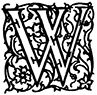henever one body exerts a force on another, the second body exerts on the first a force of equal magnitude in the opposite direction.

Forces occur in pairs. If you push on something, it pushes back just as hard. Since it is pushing 'back', your force on the object and the object's force on you are in opposite directions.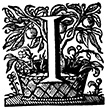f object A exerts a force F on object B, then object B exerts a force -F on object A, equal in magnitude but opposite in direction to F.

Object A pushes on object B with a force named F. Object B pushes on object A with a force named -F. Both forces are called F, so they have the same size. One force, though, has a negative sign while the other does not. This means that the forces are in opposite directions.henever one object exerts a force on a second object, the second exerts an equal and opposite force on the first.

Again, when two objects interact and push on each other, one object can not push harder or lesser than the other. They push on each other equally. The directions of the two pushes, though, are in opposite directions.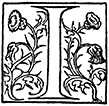f two bodies interact, the magnitude of the force exerted on object 1 by object 2 is equal to the magnitude of the force simultaneously exerted on object 2 by object 1, and these two force are opposite in direction.

The word magnitude means size. So this statement says that interacting bodies place forces on each other that are equal in size and opposite in direction.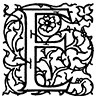very action is attended by an equal and opposite reaction.

These are very close to Newton's actual words. It is often slightly simplified as 'For every action there is an equal and opposite reaction.'

Often it is incorrectly understood that 'action' and 'reaction' mean force, and that is why one can then say 'For every force there is an equal and opposite force.'

However, 'action' and 'reaction' do not exactly translate to 'force'. An action, and also a reaction, is a change in momentum. So, what this statement actually means is that for every change in momentum there is an equal and opposite change in momentum.

Now, it is true that when changes in momentum are equal and opposite, then the forces between the interacting objects are equal and opposite. That, though, does not mean that 'action' and 'reaction' both mean 'force'. So, although Newton's second law is often stated as 'For every force there is an equal and opposite force', he was actually discussing changes in momentum.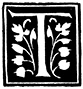o every action there is always opposed an equal reaction.

Again, like in the statement above, 'action' and 'reaction' each mean a change in momentum. To say that these changes are 'opposed' means that that if one change in momentum is positive, the other is negative. To say that these changes are 'equal' means that they have the same size.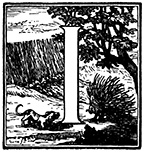f one body exerts a force on another, there is an equal and opposite force, called a reaction, exerted on the first body by the second.

Again, this statement says that interacting bodies push equally and oppositely on each other. Note here that the 'opposite force' is 'called a reaction'. Actually, when Newton used the word 'reaction' he was speaking of a change in momentum, not a force.

Custom Search